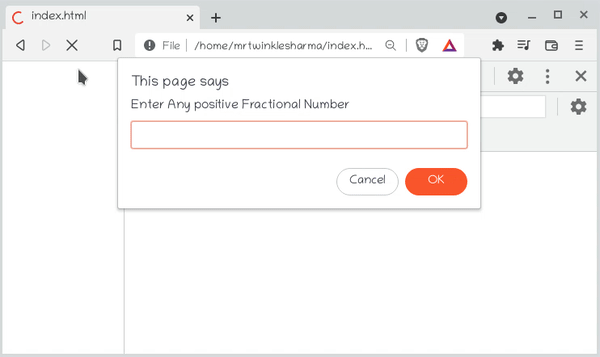GFG App
Open AppBrowser
Continue

# What is (~~) “double tilde” operator in JavaScript ?

This is a special kind of operator in JavaScript. To understand the double tilde operator, first, we need to discuss the tilde operator or Bitwise NOT. The (~) tilde operator takes any number and inverts the binary digits, for example, if the number is (100111) after inversion it would be (011000). So if we think closely it can be noticed that after inverting a number twice it will be the same as before, for example, invert the (011000) again and it would become (100111) as it was earlier.

We can reach the number from where we have started after using the double tilde (~~) operator, then what is the use of this overhead? The concept is while inverting the number this (~) operator converts them to a 32-bit signed integer which doesn’t keep fractional values. And when we invert that signed integer again, it results in a normal inversion, and due to this, the number either becomes the floor (an integer less than or equal to the number) of the original number or ceil (an integer greater than or equal to the number) of the original number.

Below are the usages of this operator.

Usage:

1. It is used as an alternative for calculating floor instead of using Math.floor(), but the condition given number should be positive.
2. It is used as an alternative for calculating the integer part of a fractional number instead of using Math.trunc(), but the condition is that the given number should be negative.
3. It is used as an alternative for calculating the ceil instead of using Math.ceil(), but the condition that is given the number should be negative.
4. It can be used to convert false values like undefined to zero so it becomes useful when we want to create a counter on such values without facing any errors.

Example 1: The following example demonstrates both of the above functions. First of all, we have declared a variable, and the prompt is taking input from the user. We are using the ~~ operator which will calculate the floor of the number, If the entered number was positive, or it calculates ceil of the number if the entered number was negative.

## JavaScript

 ``

Output:Example 2: In this example, we will create a counter to check the number of duplicates of a particular type in an array using tilde

## Javascript

 `let number=[1, 2, 3, 1, 1, 7]; ` `let histogram={}; ` `number.forEach( num => histogram[num] = ~~histogram[num] + 1 ); ` `console.log(``"histogram=="` `+ histogram);`

Output:

`dupCount==3`

Explanation: We do not get any error of undefined while using the counter as the double tilde operator converts the previously undefined value i.e. the value of the undefined array element to zero and it can be used to increment the counter.

My Personal Notes arrow_drop_up